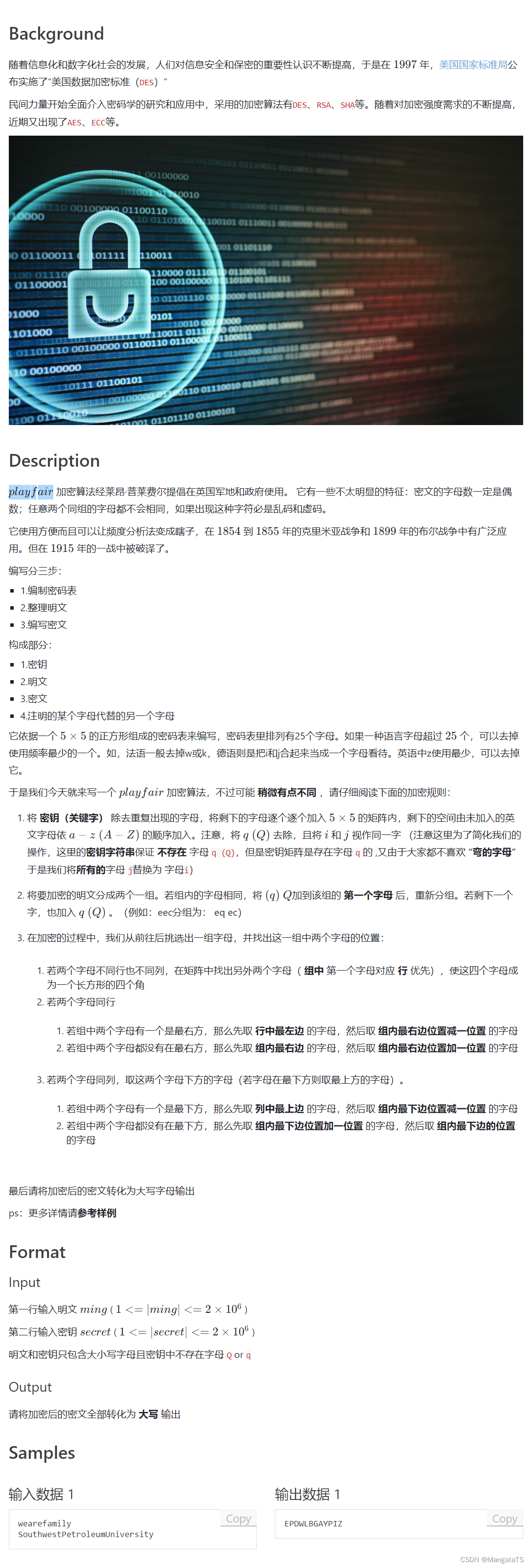# Playfair加密算法¶

## 一、简介¶

$$playfair$$ 加密算法经莱昂·普莱费尔提倡在英国军地和政府使用。 它有一些不太明显的特征：密文的字母数一定是偶数；任意两个同组的字母都不会相同，如果出现这种字符必是乱码和虚码。

• 1.编制密码表
• 2.整理明文
• 3.编写密文

• 1.密钥
• 2.明文
• 3.密文
• 4.注明的某个字母代替的另一个字母

## 二、原理¶

1. 密钥（关键字） 除去重复出现的字母，将剩下的字母逐个逐个加入 $$5 \times 5$$ 的矩阵内，剩下的空间由未加入的英文字母依 $$a-z \ (A-Z)$$ 的顺序加入。注意，将 $$q \ (Q)$$ 去除，且将 $$i$$$$j$$ 视作同一字 （注意这里为了简化我们的操作，这里的 密钥字符串 保证 不存在 字母 q (Q)，但是密钥矩阵是存在字母 q 的 ,为了统一流程是我们将所有的字母 j替换为 字母i
2. 将要加密的明文分成两个一组。若组内的字母相同，将 $$(q)\ Q$$加到该组的 第一个字母 后，重新分组。若剩下一个字，也加入 $$q\ (Q)$$ （例如：eec分组为： eq ec
3. 在加密的过程中，我们从前往后挑选出一组字母，并找出这一组中两个字母的位置：
4. 若两个字母不同行也不同列，在矩阵中找出另外两个字母（ 组中 第一个字母对应 优先），使这四个字母成为一个长方形的四个角
5. 若两个字母同行，那么就分别选取两个字母右边的第一个字母作为加密的的词，如果有一个字母在在字母表的 最右边 那么它的下一个字母就是该行的 第一个字母（解密反向）
6. 若两个字母同列，那么就分别选取两个字母下面的第一个字母作为加密的词，如果有一个字母在字母表的 最下面 那么它的下一个字母就是该列的 第一个字母 （解密反向）

$\begin{bmatrix} P \ L \ A \ Y \ F\\ I/J \ R \ S \ D \ G \\ M \ C \ H \ E B \\ K \ N \ O \ Q \ T \\ U \ V \ W \ X \ Z \\ \end{bmatrix}$

|明文分组|pl|ay| fa|ir|ci|ph|er| |---|---|---|---|---|---|---|---| |加密密文|LA|YF|PY|RS|MR|AM|CD|

P = wearefamily K = SouthwestPetroleumUniversity 得到： C = EPDWLBGAYPIZ

P = Hidethegoldinthetreestump K = Playfairexample 得到： C = BMODZBXDNABEKUDMUIXOMOUVIF

## 三、例题¶## 四、代码¶

#include<bits/stdc++.h>
using namespace std;
#define endl "\n"
char key;
map<char,bool> vis;//标记这个字母是否被用过
map<char,pair<int,int>> pos;//每个字母对应的矩阵位置
unordered_set<char> Set;

string Playfair(string ming,string secret){
int n = secret.size();
int x = 0,y = 0;
for(int i = 0;i < n; ++i) {
char c = secret[i];
if(c == 'J') c = 'I';
if(vis[c] == false) {
vis[c] = true;
pos[c]={x,y};
key[x][y] = c;
x += (++y/5);
y %= 5;
}
}
vis['J'] = true;
for(int i = 0;i < 26; ++i) {
char c = char(i + 'A');
if(!vis[c]) {
vis[c] = true;
pos[c]={x,y};
key[x][y] = c;
x += (++y/5);
y %= 5;
}
}
//以上部分是通过密钥构造字母矩阵
vector<pair<char,char>> V;
int loc = 0;
n = ming.size();
while(true){
if(loc + 1 >= n) break;
//将两者放在一组
if(ming[loc] == 'J') ming[loc] = 'I';
if(ming[loc+1] == 'J') ming[loc+1] = 'I';
if(ming[loc] == ming[loc+1])
V.push_back({ming[loc],'Q'}),loc++;
else
V.push_back({ming[loc],ming[loc+1]}),loc+=2;
}
//如果出来的时候发现还有一位明文，那么补齐q
if(loc == n-1)
V.push_back({ming[loc],'Q'});
string ans = "";
int m = V.size();
for(int i = 0;i < m; ++i) {
char a = V[i].first,b = V[i].second;
int xa = pos[a].first,ya = pos[a].second;
int xb = pos[b].first,yb = pos[b].second;
if(xa != xb && ya != yb) {
ans += key[xa][yb];
ans += key[xb][ya];

} else {
if(xa == xb) {//如果同一行
ans += key[xa][(ya + 1) % 5];
ans += key[xb][(yb + 1) % 5];
}
else if(ya == yb) {//如果同一列
ans += key[(xa + 1) % 5][ya];
ans += key[(xb + 1) % 5][yb];
}
}
}

return ans;
}

int main()
{
ios::sync_with_stdio(0);cin.tie(0);cout.tie(0);
string ming,secret;//不超过 1e5吧
cin>>ming>>secret;
transform(ming.begin(),ming.end(),ming.begin(), ::toupper);
transform(secret.begin(),secret.end(),secret.begin(), ::toupper);
string ans = Playfair(ming,secret);
cout<<ans<<endl;
return 0;
}